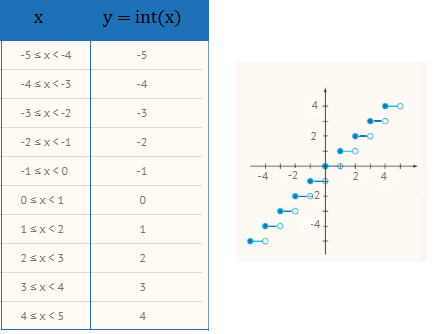# Floor Function and Ceiling Function: Simple Definition, Table & Graph

Types of Functions > Floor Function and Ceiling Function

## What is a Floor Function?

The floor function (also called the greatest integer function) rounds down a value to the closest integer less than or equal to that value. For example:

• Floor (9.9) = 6
• Floor (-9.5) = -10
• Floor (π) = 3

The floor function is similar to the ceiling function, which rounds up. Essentially, they are the reverse of each other.

The floor function is a type of step function where the function is constant between any two integers. The table below shows values for the function from -5 to 5, along with the corresponding graph:In computing, many languages include the floor function. For example:

• Java includes FLOOR (as well as CEIL).
• In BASIC, the floor function is called INT.
• For Mathematica, use Floor[x].

Other programming languages use the INTEGER PART function (int(x)).
You may want to use these functions to truncate numbers with many decimal places, like π.

## What is a Ceiling Function?

The ceiling function (also called the least integer function) rounds up a value to the closet integer greater than or equal to that value. For example:

• Ceiling (8.9) = 9
• Ceiling (-9.5) = -9
• Ceiling (π) = 4

It’s similar to the floor function, which rounds down.

In computer science, a ceiling function takes one numerical argument and returns the smallest integer that is greater than or equal to the argument’s numeric value.

## Notation for Floor Function and Ceiling Function

The name and notation for the floor function was first described by K. E. Iverson (in Graham et. al, 1990); It is usually written with square brackets missing their tops:
⌊ x ⌋.
You may also see the notation in some texts as [x], which can be confusing (is it a floor, a ceiling, or both?).

The ceiling function has similar notation, except that the “floor” part of the bracket is missing: ⌈ x ⌉. This is very similar to square brackets [ x ], but note that the missing bottom part. The floor function has similar notation, except that the “ceiling” part of the bracket is missing: ⌊ x ⌋. Sometimes, you might see other notation, including:

• Reversed boldface brackets:] x [.
• Reversed plain brackets: ] x [.

## Floor Function and Ceiling Function Properties

The floor function and ceiling function ⌊ x ⌋ share a few properties.

1. ⌊ x + 1 ⌋ = ⌊ x ⌋ + 1.
2. ⌈ x + 1 ⌉ = ⌈ x ⌉ + 1.
3. ⌈ x ⌉ = ⌊ x ⌋ if and only if x ∈ ℤ.
4. ⌈ x ⌉ = ⌊ x ⌋ + 1 if and only if x ∈̷ ℤ.
5. ⌊ x ⌋ = ⌈ x ⌉ + 1 if and only if x ∈̷ ℤ.

Where:

• ⌈ x ⌉ = ceiling function,
• ⌊ x ⌋ = flooor function,
• ℤ = “the set of all integers”,
• ∈ = “in the set,”
• ∈̷ = “not in the set.”

## Real Life Examples

A real life example of a ceiling function can be found in postage stamps. In 2019, the cost of a first class postage stamp, up to one ounce, is 55 cents. Each additional ounce costs an extra 15 cents, up to 3 ounces. Therefore, you can say that the price(p) of a first class letter is:
p(x) = 0.55 + 0.15⌈ x – 1 ⌉, 0 < x ≤ 3.

Example: The cost of mailing a first class envelope weighing 2.8 ounces is:
p(2.8) = 0.55 + 0.15⌈ 2.8 – 1 ⌉ = 0.55 + 0.15⌈ 1.8 ⌉ = 0.55 + 0.15(2) = 0.85 (85 cents).

## References

Hein, J. (2003). Discrete Mathematics. Jones & Bartlett Learning. Retrieved November 21, 2019 from: https://books.google.com/books?id=qLNfpb1hBWwC
Koshy, T. Elementary Number Theory with Applications. Retrieved November 21, 2019 from: https://books.google.com/books?id=-9pg-4Pa19IC
Koshy, T. Discrete Mathematics with Applications.

CITE THIS AS:
Stephanie Glen. "Floor Function and Ceiling Function: Simple Definition, Table & Graph" From StatisticsHowTo.com: Elementary Statistics for the rest of us! https://www.statisticshowto.com/floor-function-and-ceiling-function/